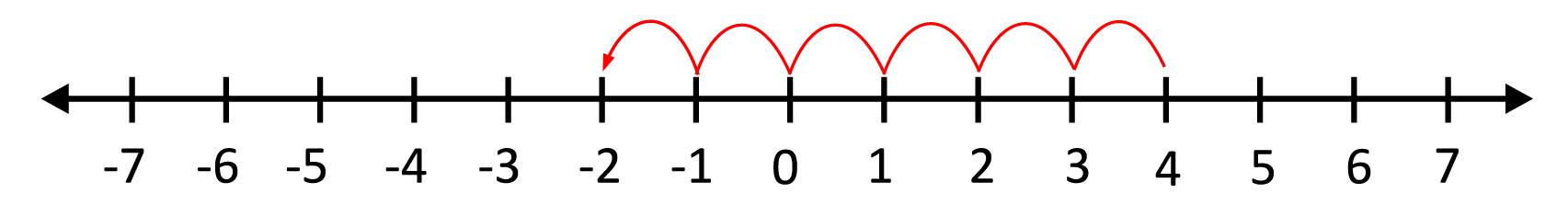### Adding two numbers with different signs:

To add numbers with different signs, we subtract the two numbers, and the answer gets the sign of the number with the largest absolute value.

Example:

Find the sum of 7 and -9. Since the two numbers have different signs, we subtract the two numbers first.

$$9-7=2$$

The absolute value of 7 is 7, the absolute value of -9 is 9. Since 9 has the largest absolute value and it is negative, the answer will get the sign of the larger number, therefore, the answer is -2.

$$7 + (-9) = -2$$

Example:

Find the sum of 14 and -6. Since the numbers have different signs, we subtract the two numbers to get 8. Then we take the absolute value of each number. The absolute value of 14 is 14, the absolute value of -6 is 6. Since 14 is the larger number and it is positive, the answer is positive, therefore, the sum of 14 and -6 is 8.

$$14+(-6)=8$$

### Adding two numbers with the same sign:

To add numbers with the same signs, we add the two numbers, and the answer gets the sign of both numbers.

Example:

Find the sum of -4 and -8. Since both numbers have the same sign, we start by adding the absolute value of the two numbers, which is $$4+8=12$$. Since both 4 and 8 are negative, the answer will also be negative. Therefore, the sum is -12.

$$(-4)+(-8)=-12$$

Subtracting a positive number is the same as adding a negative number. Let us observe this on the number line. We will look at the problem 4 – 6. We start at 4 on the number line, since we are subtracting, we will move 6 to the left, which lands us on -2.

$$4–6=-2$$Now let us look at 4 + (-6). We can solve this using our rules for adding numbers with different signs. Since the two numbers have different signs, we subtract the two and the answer gets the sign of the number with the larger absolute value.

$$6-4=2$$

The absolute value of 4 is 4, the absolute value of -6 is 6, therefore, the answer gets the sign of 6, which is negative.

$$4+(-6)=-2$$

### The Commutative Property of Addition:

The commutative property of addition states that changing the order of the two numbers you are adding does not change the results. For example, $$2+3$$ is the same as $$3+2$$. This remains true if one of the numbers is negative. $$5+(-4)$$ will give the same answer as $$(-4)+5$$.

## Adding Signed Numbers Sample Questions

Here are a few sample questions going over adding signed numbers.

Question #1:

Find the sum: $$14+(-22)$$

$$-36$$
$$-8$$
$$8$$
$$36$$

When adding two numbers with different signs, we start by subtracting the two numbers.
$$22-14=8$$

The answer gets the sign of the number with the larger absolute value. The absolute value of $$14$$ is $$14$$, the absolute value of $$-22$$ is $$22$$. Since $$22$$ is larger than $$14$$, the answer will have a negative sign.
$$14+(-22)=-8$$

Question #2:

What is the sum of $$-18+(-11)$$?

$$-29$$
$$-7$$
$$7$$
$$29$$

When adding two numbers with the same sign, we add the two numbers, and the answer gets the sign of the two numbers. Therefore, we start by adding $$18$$ and $$11$$.
$$18+11=29$$

Since they are both negative, the answer will also be negative.
$$-18+(-11)=-29$$

Question #3:

Find the sum of $$-25$$ and $$44$$.

$$-69$$
$$-19$$
$$19$$
$$69$$

When adding two numbers with different signs, we start by subtracting the two numbers. The difference between $$44$$ and $$25$$ is $$19$$. Then we take the absolute value of each term to see which is the largest. The absolute value of $$-25$$ is $$25$$, the absolute value of $$44$$ is $$44$$. Since $$44$$ is the larger number and has a positive sign, the answer will also have a positive sign.

Question #4:

What is $$85–98$$?

$$-183$$
$$-13$$
$$13$$
$$183$$

Since subtraction is the same as adding a negative, we can change the problem to $$85+(-98)$$. Now this is a problem we are used to seeing and we can use the rules for adding signed numbers. Since the numbers have different signs, we subtract the two and the answer will get the sign of the number with the larger absolute value. The difference between $$85$$ and $$98$$ is $$13$$. The absolute value of $$85$$ is $$85$$, the absolute value of $$-98$$ is $$98$$. Since $$98$$ is larger than $$85$$ and it is a negative number, the answer will be negative.

Question #5:

What is the sum of $$-66$$ and $$-78$$?

$$12$$
$$144$$
$$-12$$
$$-144$$
When adding signed numbers where both numbers have the same sign, we add the two numbers, and the answer gets the sign of both numbers. The sum of $$66$$ and $$78$$ is $$144$$. Since both numbers are negative, the answer gets the sign of both numbers and will be $$-144$$.